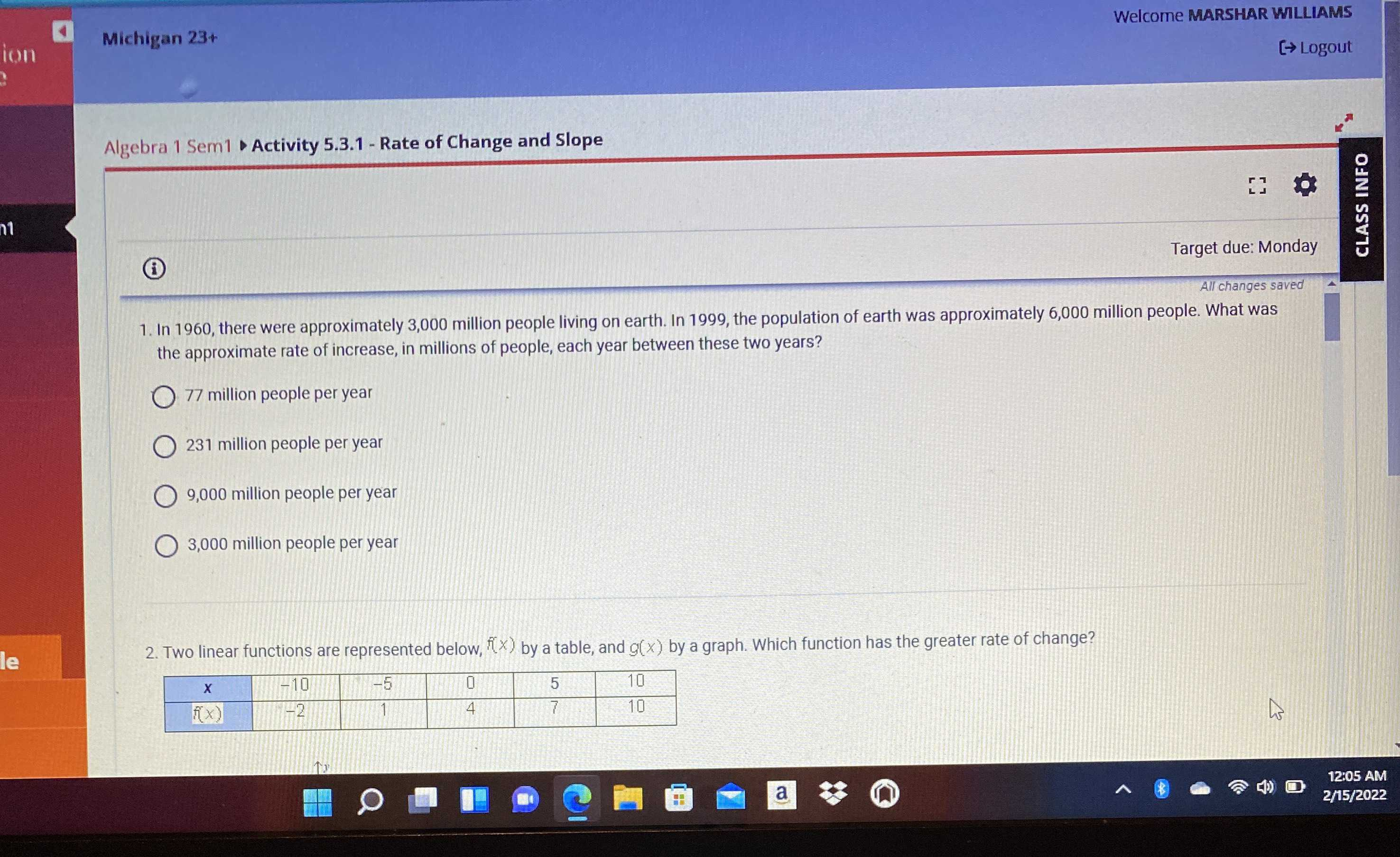### ¿Todavía tienes preguntas de matemáticas?

Pregunte a nuestros tutores expertos
Algebra
Pregunta1. In $$1960$$ , there were approximately $$3,000$$ million people living on earth. In $$1999$$ , the population of earth was approximately $$6,000$$ million people. What was the approximate rate of increase, in millions of people, each year between these two years?

$$77$$ million people per year

$$231$$ million people per year

$$9,000$$ million people per year

$$3,000$$ million people per year